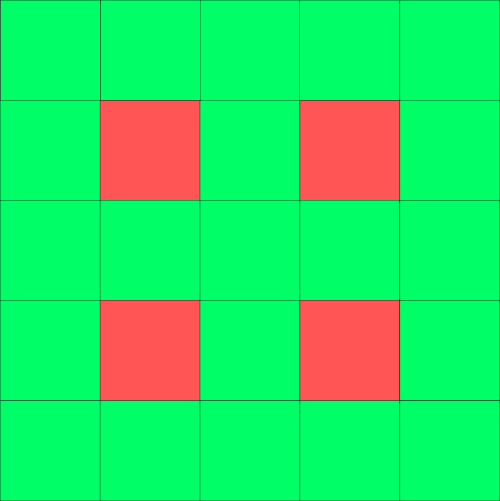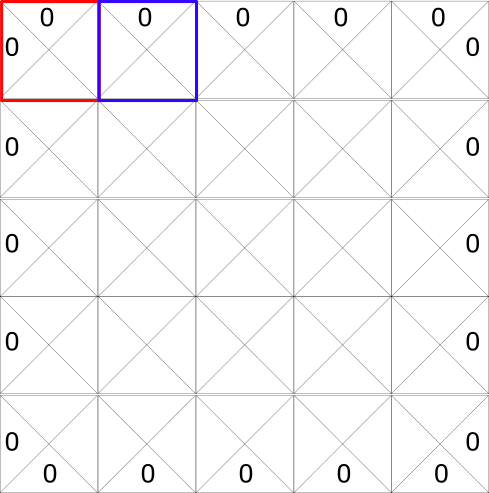by Thomas Simonini

# Diving deeper into Reinforcement Learning with Q-Learning

Today we’ll learn about Q-Learning. Q-Learning is a value-based Reinforcement Learning algorithm.

• What Q-Learning is
• How to implement it with Numpy

### The big picture: the Knight and the Princess

Let’s say you’re a knight and you need to save the princess trapped in the castle shown on the map above.

You can move one tile at a time. The enemy can’t, but land on the same tile as the enemy, and you will die. Your goal is to go the castle by the fastest route possible. This can be evaluated using a “points scoring” system.

• You lose -1 at each step (losing points at each step helps our agent to be fast).
• If you touch an enemy, you lose -100 points, and the episode ends.
• If you are in the castle you win, you get +100 points.

The question is: how do you create an agent that will be able to do that?

Here’s a first strategy. Let say our agent tries to go to each tile, and then colors each tile. Green for “safe,” and red if not.The same map, but colored in to show which tiles are safe to visit.

Then, we can tell our agent to take only green tiles.

But the problem is that it’s not really helpful. We don’t know the best tile to take when green tiles are adjacent each other. So our agent can fall into an infinite loop by trying to find the castle!

### Introducing the Q-table

Here’s a second strategy: create a table where we’ll calculate the maximum expected future reward, for each action at each state.

Thanks to that, we’ll know what’s the best action to take for each state.

Each state (tile) allows four possible actions. These are moving left, right, up, or down.0 are impossible moves (if you’re in top left hand corner you can’t go left or up!)

In terms of computation, we can transform this grid into a table.

This is called a Q-table (“Q” for “quality” of the action). The columns will be the four actions (left, right, up, down). The rows will be the states. The value of each cell will be the maximum expected future reward for that given state and action.

Each Q-table score will be the maximum expected future reward that I’ll get if I take that action at that state with the best policy given.

Why do we say “with the policy given?” It’s because we don’t implement a policy. Instead, we just improve our Q-table to always choose the best action.

Think of this Q-table as a game “cheat sheet.” Thanks to that, we know for each state (each line in the Q-table) what’s the best action to take, by finding the highest score in that line.

Yeah! We solved the castle problem! But wait… How do we calculate the values for each element of the Q table?

To learn each value of this Q-table, we’ll use the Q learning algorithm.

### Q-learning algorithm: learning the Action Value Function

The Action Value Function (or “Q-function”) takes two inputs: “state” and “action.” It returns the expected future reward of that action at that state.

We can see this Q function as a reader that scrolls through the Q-table to find the line associated with our state, and the column associated with our action. It returns the Q value from the matching cell. This is the “expected future reward.”

But before we explore the environment, the Q-table gives the same arbitrary fixed value (most of the time 0). As we explore the environment, the Q-table will give us a better and better approximation by iteratively updating Q(s,a) using the Bellman Equation (see below!).

#### The Q-learning algorithm Process

Step 1: Initialize Q-values
We build a Q-table, with m cols (m= number of actions), and n rows (n = number of states). We initialize the values at 0.

Step 2: For life (or until learning is stopped)
Steps 3 to 5 will be repeated until we reached a maximum number of episodes (specified by the user) or until we manually stop the training.

Step 3: Choose an action
Choose an action a in the current state s based on the current Q-value estimates.

But…what action can we take in the beginning, if every Q-value equals zero?

That’s where the exploration/exploitation trade-off that we spoke about in the last article will be important.

The idea is that in the beginning, we’ll use the epsilon greedy strategy:

• We specify an exploration rate “epsilon,” which we set to 1 in the beginning. This is the rate of steps that we’ll do randomly. In the beginning, this rate must be at its highest value, because we don’t know anything about the values in Q-table. This means we need to do a lot of exploration, by randomly choosing our actions.
• We generate a random number. If this number > epsilon, then we will do “exploitation” (this means we use what we already know to select the best action at each step). Else, we’ll do exploration.
• The idea is that we must have a big epsilon at the beginning of the training of the Q-function. Then, reduce it progressively as the agent becomes more confident at estimating Q-values.

Steps 4–5: Evaluate!
Take the action a and observe the outcome state s’ and reward r. Now update the function Q(s,a).

We take the action a that we chose in step 3, and then performing this action returns us a new state s’ and a reward r (as we saw in the Reinforcement Learning process in the first article).

Then, to update Q(s,a) we use the Bellman equation:

The idea here is to update our Q(state, action) like this:

``New Q value =    Current Q value +    lr * [Reward + discount_rate * (highest Q value between possible actions from the new state s’ ) — Current Q value ]``

Let’s take an example:

• One cheese = +1
• Two cheese = +2
• Big pile of cheese = +10 (end of the episode)
• If you eat rat poison =-10 (end of the episode)

Step 1: We init our Q-table

Step 2: Choose an action
From the starting position, you can choose between going right or down. Because we have a big epsilon rate (since we don’t know anything about the environment yet), we choose randomly. For example… move right.

We found a piece of cheese (+1), and we can now update the Q-value of being at start and going right. We do this by using the Bellman equation.

Steps 4–5: Update the Q-function

• First, we calculate the change in Q value ΔQ(start, right)
• Then we add the initial Q value to the ΔQ(start, right) multiplied by a learning rate.

Think of the learning rate as a way of how quickly a network abandons the former value for the new. If the learning rate is 1, the new estimate will be the new Q-value.

Good! We’ve just updated our first Q value. Now we need to do that again and again until the learning is stopped.

### Implement a Q-learning algorithm

We made a video where we implement a Q-learning agent that learns to play Taxi-v2 with Numpy.

Now that we know how it works, we’ll implement the Q-learning algorithm step by step. Each part of the code is explained directly in the Jupyter notebook below.

You can access it in the Deep Reinforcement Learning Course repo.

Or you can access it directly on Google Colaboratory:

### A recap…

• Q-learning is a value-based Reinforcement Learning algorithm that is used to find the optimal action-selection policy using a q function.
• It evaluates which action to take based on an action-value function that determines the value of being in a certain state and taking a certain action at that state.
• Goal: maximize the value function Q (expected future reward given a state and action).
• Q table helps us to find the best action for each state.
• To maximize the expected reward by selecting the best of all possible actions.
• The Q come from quality of a certain action in a certain state.
• Function Q(state, action) → returns expected future reward of that action at that state.
• This function can be estimated using Q-learning, which iteratively updates Q(s,a) using the Bellman Equation
• Before we explore the environment: Q table gives the same arbitrary fixed value → but as we explore the environment → Q gives us a better and better approximation.

That’s all! Don’t forget to implement each part of the code by yourself — it’s really important to try to modify the code I gave you.

Try to add epochs, change the learning rate, and use a harder environment (such as Frozen-lake with 8x8 tiles). Have fun!

Next time we’ll work on Deep Q-learning, one of the biggest breakthroughs in Deep Reinforcement Learning in 2015. And we’ll train an agent that that plays Doom and kills enemies!